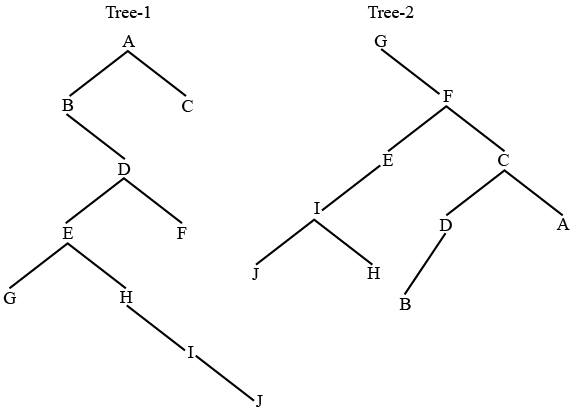# ISRO Scientist or Engineer Computer Science 2018

Instructions

For the following questions answer them individually

Question 11

# In unit testing of a module, it is found that for a set of test data, at the maximum 90% of the code alone weretested with the probability of success 0.9. The reliability of the module isQuestion 12

# In a file which contains 1 million records and the order of the tree is 100, then what is the maximum number of nodesto be accessed if B+ tree index is used?Question 13

# A particular disk unit uses a bit string to record the occupancy or vacancyof its tracks, with 0 denoting vacant and I for occupied. A 32-bit segment of this string has hexadecimal value D4FE2003. The percentage of occupied tracks for the corresponding part of the disk, to the nearest percentage isQuestion 14

# Which of the following is dense index?Question 15

# In E-R model,Y is the dominant entity and X is subordinate entityQuestion 16

# Of the following, which best characterizes computers that use memory-mapped I/O?Question 17

# Of the following sorting algorithms, which has a running time thatis least dependent on the initial ordering of the input?Question 18

# Processes P1 and P2 have a producer-consumer relationship, communicating by the use of a set of shared buffers.P1: repeat Obtain an empty buffer Fill it Return a full buffer foreverP2: repeat Obtain a full buffer Empty it Return an empty buffer foreverIncreasing the number of buffersis likely to do which of the following?I. Increase the rate at which requests are satisfied (throughput)II. Decrease the likelihood of deadlockIII. Increase the ease of achieving a correct implementationQuestion 19

# A doubly linked list is declared asstruct Node { int Value ; struct Node *Fwd ; struct Node *Bwd ;};Where Fwd and Bwd represent forward and backward link to the adjacent elements of the list. Which of the following segments of code deletes the node pointed to by X from the doublylinked list, if it is assumedthat X points to neither the first nor the last node of the list?Question 20

# If Tree-1 and Tree-2 are the trees indicated below :Which traversals of Tree-1 and Tree-2, respectively, will produce the same sequence?OR